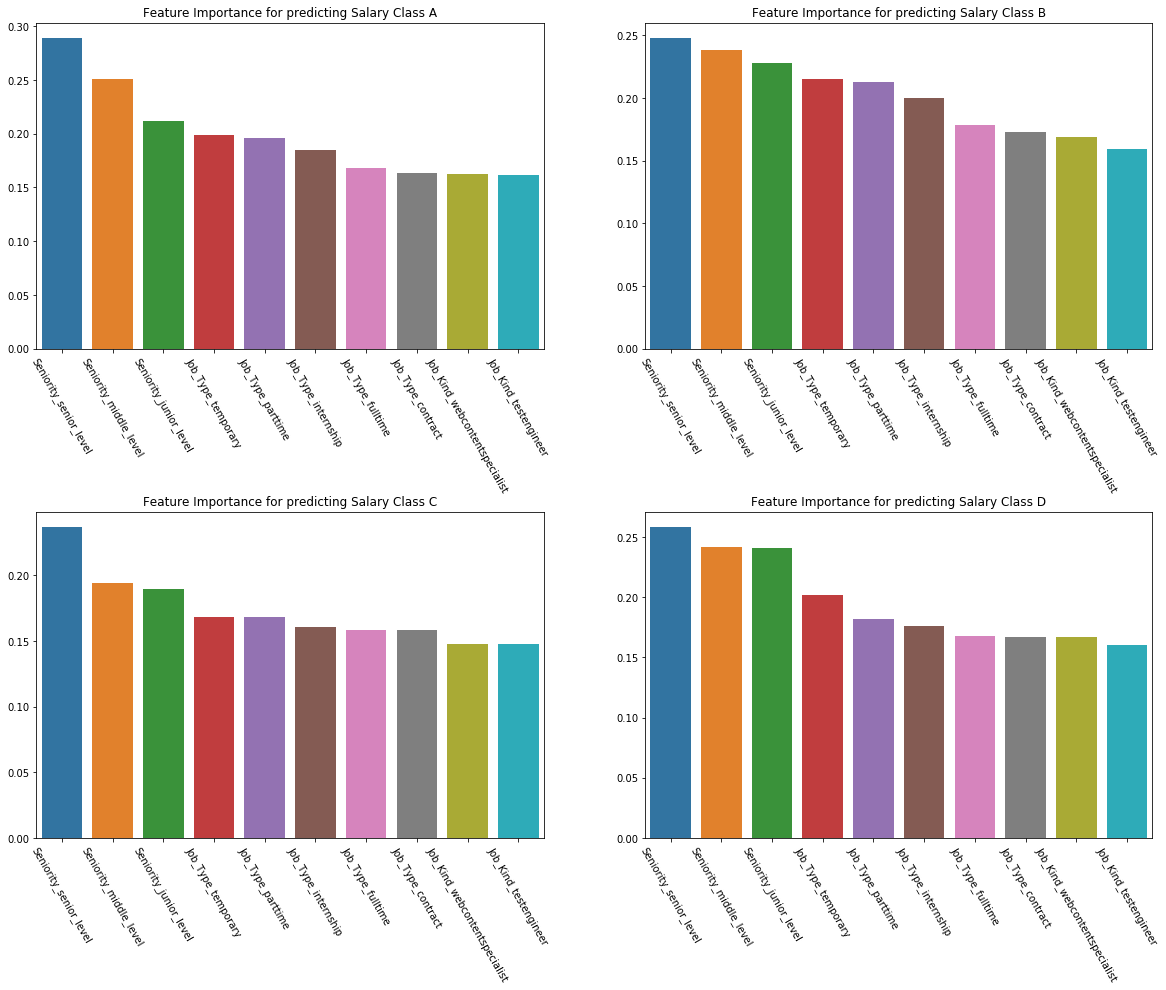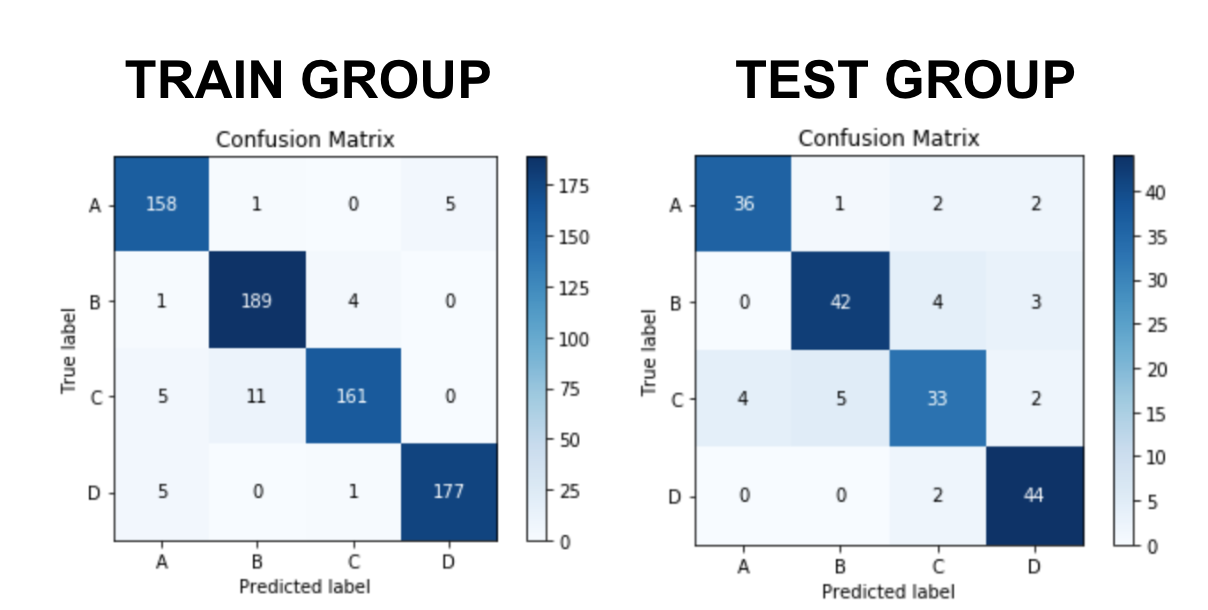# A Classification Project in Machine Learning: a gentle step-by-step guide

Classification is a core technique in the fields of data science and machine learning that is used to predict the categories to which data should belong. Follow this learning guide that demonstrates how to consider multiple classification models to predict data scrapped from the web.Classification is one of the main kinds of projects you can face in the world of Data Science and Machine Learning. Here is Wikipedia’s definition:

Classification is the problem of identifying to which of a set of categories (sub-populations) a new observation belongs, on the basis of a training set of data containing observations (or instances) whose category membership is known. Examples are assigning a given email to the “spam” or “non-spam.”

For this post, I’ll go through a project from my General Assembly’s Immersive in Data Science. In this, I explored different machine learning classification models to predict four salary categories for Data Science job posts using publications from Indeed.co.uk:

• Salary below percentile 25%
• Salary between percentile 25 and 50%
• Salary between percentile 50 and 75%
• Salary above percentile 75%

We won’t be able to go through every single aspect of the project, but be aware that the entire repository is available on my GitHub profile.

### First Stage: Scraping and Cleaning

First and foremost, no project will ever be anything without data. So I started by scraping Indeed.co.uk in order to obtain a list of job posts looking for ‘data scientists’ in several cities of the UK. I won’t cover how to actually do the scraping here, but I used the same techniques and tools mentioned in another post of mine: Web scraping in five minutes.

It’s worth mention though that even though web scraping is great and very useful for those working in data science, always check the completeness of your data once you finish scraping. For example, in this case, having the job post salary was, of course, key. However, not all publications on Indeed include salary, so it was necessary to scrap thousands of pages and job posts in order to have at least 1000 job posts that contain a salary.

Working with scraped data usually also involves lots of feature engineering to add some value from the data we already have. For example, for this project, I developed a ‘Seniority’ feature, which is created from the Title and Summary of each publication, using two different lists with words belonging to senior or junior levels of jobs. If any word of each level was present, either on the job title, in the summary, then the corresponding seniority level was assigned. If none of the words were in those features, the job post was assigned as a middle-level.

### Second Stage: Modelling

I started this stage exploring three different models:

• A KNN model with bagging: KNN stands for a K-Nearest Neighbours model. This works by checking the class of the nearest points to the one being predicted, in order to classify it. Combining it with bagging, we can improve the stability and accuracy while also reducing variance and helping to avoid overfitting.How? Bagging is an ensemble method — a technique that combines the predictions from multiple machine learning algorithms to make more accurate predictions than any individual model. Although it’s usually applied to decision tree methods, it can be used with any type of method.
• A Decision Tree model with boosting: in this case a decision tree works as a flowchart-like structure in which each internal node represents a “test” on an attribute (e.g., whether a coin flip comes up heads or tails), each branch represents the outcome of the test, and each leaf node represents a class label and a decision taken. The paths from the root to the leaf represent classification rules. In this model, although boosting is a very different method than bagging, it is also an ensemble method — one that works by building a model from the training data, then creating a second model that attempts to correct the errors from the first model. Models are added until the training set is predicted perfectly, or a maximum number of models are added.

Since these two models are highly dependent on the given hyperparameters, you’ll probably want to use GridSearch in order to optimize them as much as possible. GridSearch is simply a tool that trains several models looking for the best parameters from a given list of parameters and values.

So, for example, for creating a Decision Tree model with boosting and GridSearch you would take the following steps.

1. Instantiate the model

```from sklearn.tree import DecisionTreeRegressor

tree_to_boost = DecisionTreeRegressor(random_state=123)

```

2. Instantiate the ensemble method algorithm

```from sklearn.ensemble import AdaBoostClassifier

boosting_trees_model = AdaBoostClassifier(base_estimator = tree_to_boost, random_state=123)

```

3. Instantiate GridSearch and specify the parameters to be tested

When using GridSearch you can get the available parameters to be tuned just by calling get_params() over the previously instantiated model:

```tree_to_boost.get_params()

```

Remember: you can always get more detail about how to optimize any hyperparameters in Sklearn’s documentation. For example, here is the decision trees doc.

Finally, let’s import GridSearch, specify the parameters wanted and instantiate the object. Be aware that sklearn’s GridSearchCV includes the cross-validation within the algorithm, so you will have to specify the number of CV to be done too,

```from sklearn.model_selection import GridSearchCV

trees_params = {‘base_estimator__max_depth’: [80,100,120,150],
‘base_estimator__max_features’: [0.93,0.95,0.97],
‘n_estimators’: }

boosting_tree_grid = GridSearchCV(estimator=boosting_trees_modeel, param_grid=tree_params, cv=5)

```

4. Fit your combined GridSearch and check the results

Fitting the GridSearch is like fitting any model:

```boosting_tree_grid.fit(X_train, y_train)

```

Once it’s done you can check the best parameters to see if you still have an opportunity to optimize any of them. Just run the following piece of code:

```boosting_tree_grid.best_params_

```

As in any mode, you can use .score() and .predict() using the GridSearchCV object.

### Stage Three: Feature Importance

After modeling, the next stage is always analyzing how our model is performing and why it is doing what it’s doing.

However, if you’ve had the chance to work with ensemble methods, you probably already know that these algorithms are usually known as “black-box models.” These models lack explicability and interpretability since the way they usually work implies one or several layers of a machine making decisions without human supervision, apart from a group of rules or parameters set. More often than not, not even the most expert professionals in the field can understand the function that is actually created by, for example, training a neural network.

In this sense, some of the most classical machine learning models were actually better. That’s why, for the sake of this post, we’ll be analyzing the feature importance of our project using a classic Logistic Regression. However, if you’re interested in knowing how to analyze feature importance for a black-box model, in this other article of mine, I explored a tool for doing just that.

Starting from a Logistic Regression model, getting the feature importance is as easy as calling:

```logistic_regression_model.coef_[class_number]

```

A neat way of seeing the overall feature importance is by creating a DataFrame with the feature importance for each class. I like to do it using the absolute value for each feature, in order to see the absolute impact each one has in the model. However, mind that if you want to analyze specifically how each feature helps to increase or decrease the possibility of being each class, you should take the original value, whether it is negative or positive.

Remember we were trying to predict four classes, so this is how we should create the Pandas DataFrame:

```feature_importance_df = pd.DataFrame({‘feature’: logistic_regression_model.columns,

‘coefA’: np.abs(logistic_regression_model.coef_),

‘coefB’: np.abs(logistic_regression_model.coef_),

‘coefC’: np.abs(logistic_regression_model.coef_),

‘coefD’: np.abs(logistic_regression_model.coef_),})

```

We can finally put everything in plots and see how each class behaves:Even though perhaps the size of the labels doesn’t help, we can conclude from these plots that the following features of our dataset are relevant when predicting the salary category:

• Seniority: as we can see, the tree levels created impact very strongly in all categories, being the first coefficients in terms of absolute size.
• In the second place, comes the Job_Type directly scraped from Indeed
• Finally, for all the salary categories, there are two job titles scraped and cleaned from indeed: Web Content Specialist and Test Engineer.

This dataset contains hundreds of features, but it’s nice to see there’s a clear trend throughout the categories!

### Stage Four: Conclusions and Trustworthiness

In the end, the only thing left is to evaluate the performance of our model. For this, we can use several metrics. Unfortunately, going through all the possible metrics in a classification problem would be too long for this post. However, I can refer you to a very good one here in Medium, giving good details about all the key metrics. Enjoy it here.

As can read in Mohammed’s story linked above, the Confusion Matrix is the mother concept involving all the rest of the metrics. In short, it has the true labels or categories on one axis and the predicted ones on the other. In the end, we’d like to have a diagonal match in between our predictions and the real labels, with ideally zero or few cases mismatching. The metrics library from Sklearn has a beautiful and simple representation that we can plot just by feeding the algorithm with the real label and our predictions:

```from sklearn import metrics

skplt.metrics.plot_confusion_matrix(y_test, y_predictions)

plt.show()

```

Using this library, we can see in the following plots that, for this project, both the train and test groups were predicted with a solid accuracy throughout the four salary categories:One important final clarification is that, although our final model seems to be accurate, it works well to predict categories when the importance of them is equal, and we don’t have the need to ponder any class or classes.

For example, if we were creating this model for a company, for which it would be more consequential to tell a person incorrectly that they would get a low salary job than to tell a client incorrectly that they would get a high salary job, our model would struggle, since it wouldn’t be able to predict all the positive values of a class as positive, without predicting a lot of negative values incorrectly as well. In that case, we should work another way around this problem — for example, by creating a model with weighted categories.

Original. Reposted with permission.

Bio: After 5+ years of experience in eCommerce and Marketing across multiple industries, Gonzalo Ferreiro Volpi pivoted into the world of Data Science and Machine Learning, and currently works at Ravelin Technology using a combination of machine learning and human insights to tackle fraud in eCommerce.

Related: